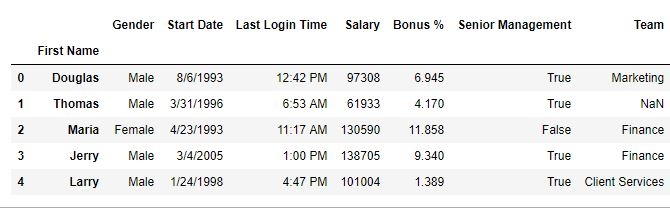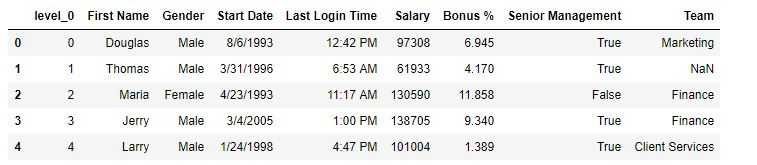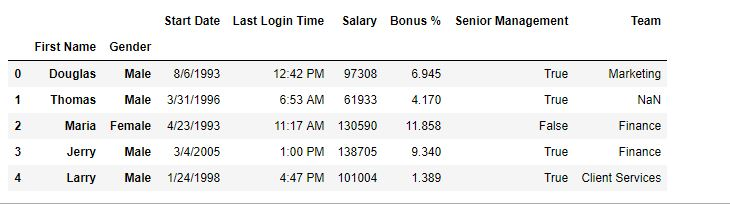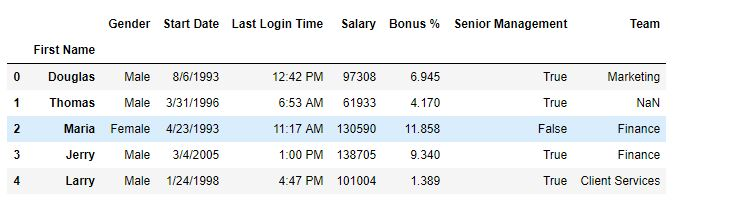Related Articles

# Python | Pandas DataFrame.reset_index()

• Difficulty Level : Easy
• Last Updated : 17 Sep, 2018

Python is a great language for doing data analysis, primarily because of the fantastic ecosystem of data-centric python packages. Pandas is one of those packages and makes importing and analyzing data much easier.

Pandas `reset_index()` is a method to reset index of a Data Frame. reset_index() method sets a list of integer ranging from 0 to length of data as index.

Syntax:
DataFrame.reset_index(level=None, drop=False, inplace=False, col_level=0, col_fill=”)

Parameters:
level: int, string or a list to select and remove passed column from index.
drop: Boolean value, Adds the replaced index column to the data if False.
inplace: Boolean value, make changes in the original data frame itself if True.
col_level: Select in which column level to insert the labels.
col_fill: Object, to determine how the other levels are named.

Return type: DataFrame

Example #1: Resetting index
In this example, to reset index, First name column have been set as index column first and then using reset index a new index have been generated.

 `# importing pandas package``import` `pandas as pd``  ` `# making data frame from csv file``data ``=` `pd.read_csv(``"employees.csv"``)``  ` `# setting first name as index column``data.set_index([``"First Name"``], inplace ``=` `True``,``                    ``append ``=` `True``, drop ``=` `True``)``  ` `# resetting index``data.reset_index(inplace ``=` `True``)``  ` `# display``data.head()`

Output:
As show in the output images, A new index label named level_0 has been generated.

Before reset –After reset –Example #2: Operation on Multi level Index
In this example, 2 columns(First name and Gender) are added to the index column and later one level is removed by using reset_index() method.

 `# importing pandas package``import` `pandas as pd``  ` `# making data frame from csv file``data ``=` `pd.read_csv(``"employees.csv"``)``  ` `# setting first name as index column``data.set_index([``"First Name"``, ``"Gender"``], inplace ``=` `True``,``                             ``append ``=` `True``, drop ``=` `True``)``  ` `# resetting index``data.reset_index(level ``=` `2``, inplace ``=` `True``, col_level ``=` `1``)``  ` `# display``data.head()`

Output:
As shown in the output image, The gender column in the index column was replaced as it’s level was 2.

Before reset –After reset –Attention geek! Strengthen your foundations with the Python Programming Foundation Course and learn the basics.

To begin with, your interview preparations Enhance your Data Structures concepts with the Python DS Course. And to begin with your Machine Learning Journey, join the Machine Learning – Basic Level Course

My Personal Notes arrow_drop_up